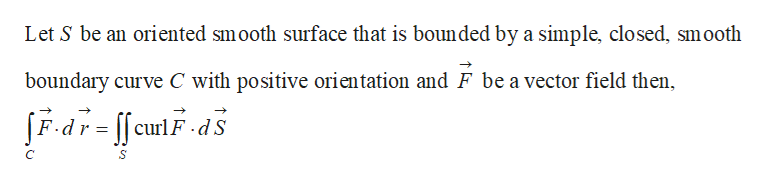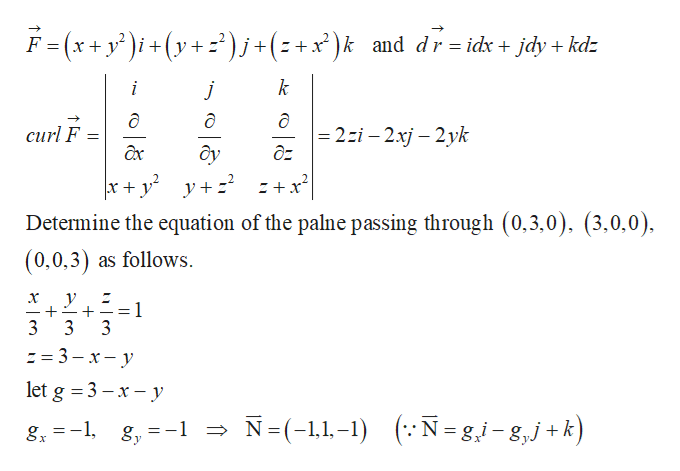# Use Stokes' Theorem to evaluate F · dr where C is oriented counterclockwise as viewed from above.F(x, y, z) = (x + y2)i + (y + z2)j + (z + x2)k,C is the triangle with vertices (3, 0, 0), (0, 3, 0), and (0, 0, 3).

Question
30 views

Use Stokes' Theorem to evaluate

F · dr

where C is oriented counterclockwise as viewed from above.

F(x, y, z) = (x + y2)i + (y + z2)j + (z + x2)k,

C is the triangle with vertices
(3, 0, 0), (0, 3, 0), and (0, 0, 3).
check_circle

Step 1

Stokes’ theoremhelp_outlineImage TranscriptioncloseLet S be an oriented smooth surface that is bounded by a simple, closed, smooth boundary curve C with positive orientation and F be a vector field then, JFdr-cuFds curl fullscreen
Step 2help_outlineImage TranscriptioncloseF(x+y') i+(y+ z )j+(z+x)k and dr =idx jdy kdz k ! - 2:і - 2xj — 2уk д2 curl F axc ôy + x Determine the equation of the palne passing through (0,3,0), (3,0,0), (0,0,3) as follows. х у 1 3 _ 3 3 = 3-x-y let g 3x-y N (-,1-1)N= gi-g,j+k) -1 =-1 gy fullscreen

### Want to see the full answer?

See Solution

#### Want to see this answer and more?

Solutions are written by subject experts who are available 24/7. Questions are typically answered within 1 hour.*

See Solution
*Response times may vary by subject and question.
Tagged in

### Math